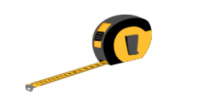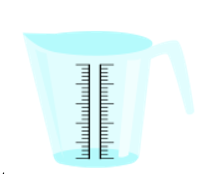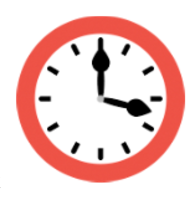Accelerate your child’s learning
Smartick is a fun way to learn math!Sep22

# Review of All Units of Measurements

In today’s post, we are going to do a review of all the units of measurement that we have seen so far in previous posts. Remember which were the main measurements? And the units we used to measure them? Let’s look at it below.

### Units of massThe main unit for measuring mass is the gram. To measure larger masses, we have multiples (decagram, hectogram, kilogram, ton …) and to measure smaller masses, there are submultiples (decigram, centigram, milligram …).

### Units of lengthThe main unit for measuring length is the meter. To measure larger lengths we have multiples (decameter, hectometer, kilometer …) and to measure smaller lengths are the submultiples (decimeter, centimeter, millimeter …).

### Units of volumeThe main unit for measuring volume is the liter. To measure larger volumes, we have multiples (decaliter, hectoliter, kiloliter …) and to measure smaller volumes, we have submultiples (deciliter, centiliter, milliliter …).

### Units of timeThe unit we are going to use as a reference to measure time is the day. To measure longer periods of time we have the week, the month, the year, etc. And to measure smaller periods of time we have the hour, the minute, the second, and so on.
Now you have a summary of the main measurements, their units and problems to understand better and learn to convert. Share it with your friends and colleagues.

If you want to continue learning more math, sign up for Smartick and try it for free.